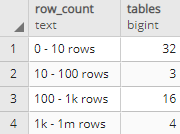## Number of tables by the number of rows in PostgreSQL database

Article for:

If you want to get an overview of the number of rows the tables contain in your database (schema), one way to count them is by intervals of rows. This query returns the number of tables grouped by the number of rows at predefined intervals.

## Query

``````select row_count,
count(*) as tables
from (
select c.relname as table_name,
n.nspname as table_schema,
case when c.reltuples > 1000000000 then '1b rows and more'
when c.reltuples > 1000000 then '1m - 1b rows'
when c.reltuples > 1000 then '1k - 1m rows'
when c.reltuples > 100 then '100 - 1k rows'
when c.reltuples > 10 then '10 - 100 rows'
else  '0 - 10 rows' end as row_count,
c.reltuples as rows
from pg_class c
join pg_namespace n on n.oid = c.relnamespace
where c.relkind = 'r'
and n.nspname not in ('pg_catalog', 'information_schema')
) itv
group by row_count
order by max(rows);
``````

## Columns

• row_count - predefined row count intervals:
• 0 - 10 rows
• 10 - 100 rows
• 100 - 1k rows
• 1k - 1m rows
• 1m - 1b rows
• 1b rows and more
• tables - number of tables whose row count falls in that interval

## Rows

• One row: represents one interval
• Scope of rows: all row count intervals that appear in the database (schema)
• Ordered by: from the smallest table row count to the largest

## Sample results

Below is a number of tables grouped by row count in predefined intervals for the pg_catalog schema.0